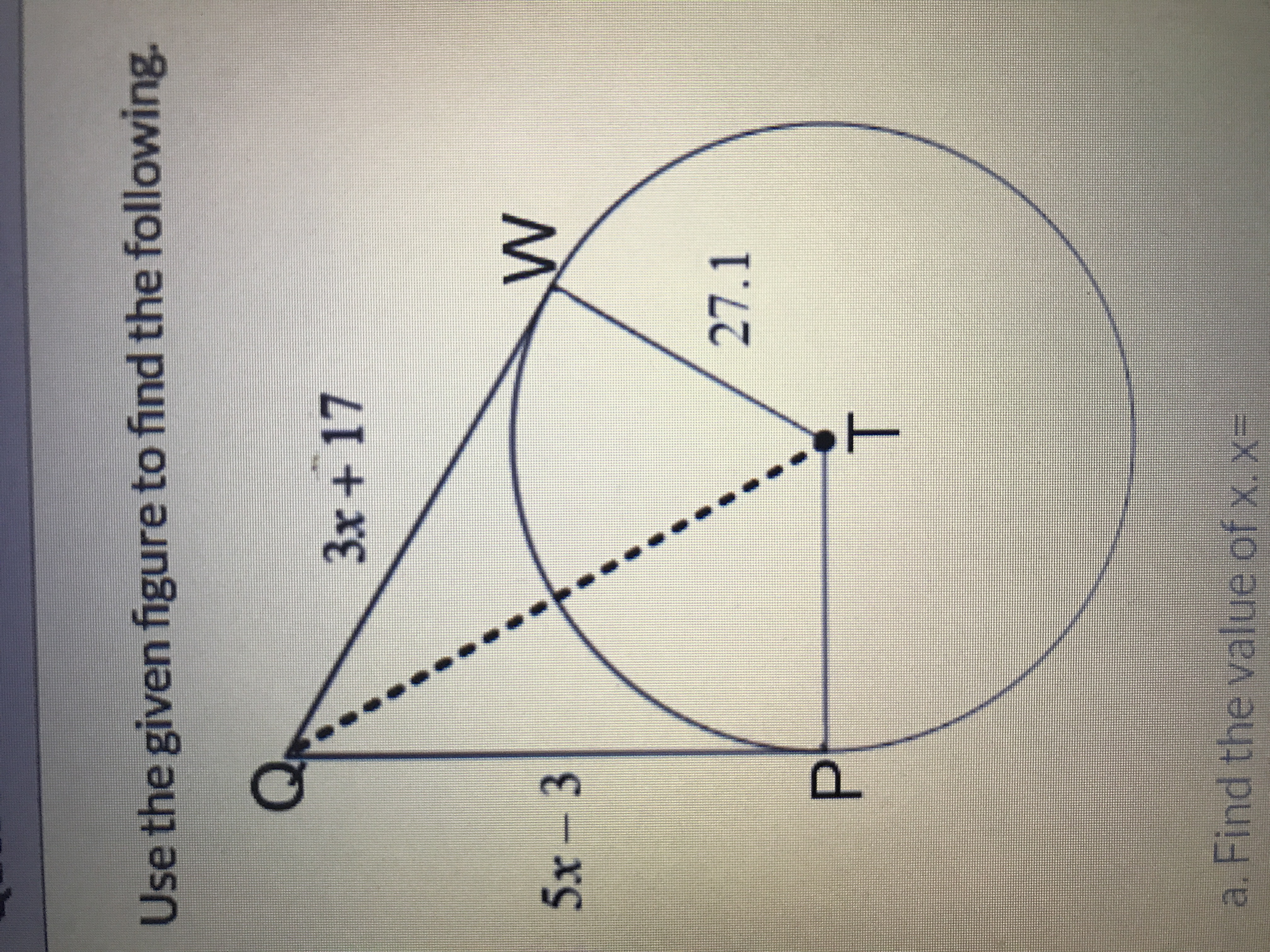# Use the given figure to find the following. 3x + 17 5x - 3 27.1 T. a. Find the value of x. x=

Question

Find the length of segment QT. Round to one decimal place.help_outlineImage TranscriptioncloseUse the given figure to find the following. 3x + 17 5x - 3 27.1 T. a. Find the value of x. x= fullscreen